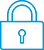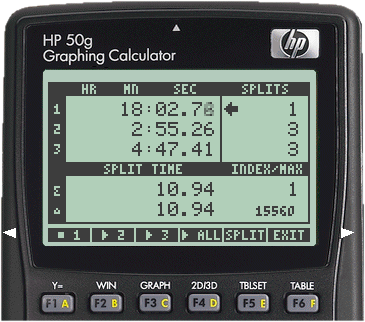cancel
Showing results for
Search instead for
Did you mean:It has been a while since anyone has replied. Simply ask a new question if you would like to start the discussion again.New member
1 0 0 0
Message 1 of 2
301
Flag Post

# Time value of moneyHP Recommended
hp 10bII

I am doing a time value of money calculation and keep getting the incorrect answer.

n = 5

PMT = -32 000

FV = -400 000

i  = 10%

p/py = 1

,PV = my answer R 527 527.10

According to memo R 369 674

What am I doing wrong?

Tags (3)
1 REPLY 1Level 6
140 139 29 60
Message 2 of 2
Flag PostHP Recommended

I have a 10bII+ instead of the 10bII, but the computation should be the same on both. The computation given the values you indicated comes out to 369673.705845 on my 10bII+(with all digits displayed), so it would appear that you may not actually have the values that you are expecting in the appropriate TVM registers.

One way to double check this before solving for PV is as follows:

• Start by clearing the TVM environment (up shift-C MEM-TVM)
• Enter your values the way you usually do, but stop just before pressing [PV] to solve for that variable
• Recall each variable in turn, and verify that the value is what you are expecting it to be

For the "standard" TVM variables (N, I/YR, PV, PMT, FV), recalling is a simple matter of pressing the [RCL] key followed by the variable key you are checking, ie. [RCL] followed by [N] will show you the current value assigned to N. Recalling P/YR is done by pressing [RCL] [down-shift] [PMT] (note that the last key in that sequence is the [PMT] key, not the [N] key).

I suspect that at least one of the variables will be different than what you are expecting, so the "fun" part of this will be to determine why the entered value isn't what you are expecting. Some possible hints:

• Negative values are entered by entering the numbers first, then press the [+/-] key at the end to change the sign (the [-] key cannot be used for this)
• P/YR is the down-shifted [PMT] key, not the down-shifted [N] key (the latter has a "*" in front to designate an implied multiplication of N by the current P/YR value)

Checking the proper BEG/END mode is a simple matter of observing whether the BEG annunciator is turned on. If you don't see "BEG" in your calculator's display near the bottom of the display, then the calculator is set to END mode. If you do see the annunciator, then the calculator is in BEGIN mode.

Remember that recalling any variables or performing any intermediate calculations leaves the calculator in a state where the current displayed value will be stored in a TVM variable if you simply press that key without the preceding [RCL]. So if you want to solve for a variable after checking these values, you may have to press that key twice in a row to cause the calculator to solve for that variable instead of simply storing a new value there.

Hope this helps!

† The opinions expressed above are the personal opinions of the authors, not of HP. By using this site, you accept the Terms of Use and Rules of Participation